Java帮帮-微信公众号Java帮帮-微信公众号将分享做到极致 支付宝小程序支付宝小程序支付也能阅读 程序员生活志-公众号程序员生活志-公众号程序员生活学习圈，互联网八卦黑料

 编程资讯
 Java
 程序员段子
 程序人生
 秒杀专栏
 名企资讯
 运维监控
 人事面试
 技术面试
 大禹编程
 UI
 SpringBoot
 SpringCloud(F版)
 SpringCloud(D版)
 spring
 Docker
 Dubbo
 IntelliJ IDEA
 Linux
 Nginx
 Tomcat
 Quartz
 微信小程序
 阿里云
 Python
 Java案例
 小程序源码
 Java源码
 手机资讯
 Sql Server
 MySQL
 MongoDB
 前端开发
 node.js
 CSS
 移动端
 jQuery
 Sublime Test
 JavaScript
 HTML
 Vue.js
 HTTPS
 分布式事物
 Redis
 JSON
 DevOps
 情感人生
 黑科技
 Oracle
 Git
 微服务
 大数据
 职业生涯
 人工智能
 Keras教程
 RocketMQ
 PyTorch教程
 Spring Security

# 第十八天 集合-泛型&list接口&set接口【悟空教程】

74# 第1章 泛型

## 1.3 泛型代码实现

### 1.3.1 含有泛型的类

• 例如，API中的ArrayList集合：

class ArrayList<E>{

public E get(int index){  }

}

• 例如，ArrayList<String> list = new ArrayList<String>();

class ArrayList<String>{

public String get(int index){  }

}

• 例如，ArrayList<Integer> list = new ArrayList<Integer>();

class ArrayList<Integer>{

public Integer get(int index){  }

}

### 1.3.2 含有泛型的方法

• 例如，API中的ArrayList集合中的方法：

public <T> T[] toArray(T[] a){  }

//该方法，用来把集合元素存储到指定数据类型的数组中，返回已存储集合元素的数组

• 例如

ArrayList<String> list = new ArrayList<String>();

String[] arr = new String;

String[] result = list.toArray(arr);

public <String> String[] toArray(String[] a){  }

• 例如

ArrayList<String> list = new ArrayList<String>();

Integer[] arr = new Integer;

Integer [] result = list.toArray(arr);

public <Integer> Integer[] toArray(Integer[] a){  }

### 1.3.3 含有泛型的接口

• 例如，API中的Iterator迭代器接口

public interface Iterator<E> {

public abstract E next();

}

1、定义类时确定泛型的类型

• 例如

public final class Scanner implements Iterator<String> {

public String next(){  }

}

2、始终不确定泛型的类型，直到创建对象时，确定泛型的类型

• 例如

Collection<String> list = new ArrayList<String>();

Iterator<String> it = list.iterator();

public interface Iterator<String> {

public abstract String next();

}

}

## 1.4 泛型通配符

? extends E代表只要是E类型的子类即可

? super E代表只要是E类型的父类即可

/*

* 泛型通配符?,代表任意的数据类型

*

* 定义：(查看ArrayList的构造方法)无法在类中使用

*

* 使用：调用方法时可以给予任意类型。参照Arraylist的构造方法

* public ArrayList(Collection<? extends E> c)

* 为了便于?的理解,我们将以上方法重写为public ArrayList(ArrayList<? extends E> c)

*

* 该方法的意思:创建集合对象A时,给于另外一个集合对象B作为参数,则创建好的集合A中包含了集合B中的元素

*

* ? extends E代表只要是E类型的子类即可

* ? super E代表只要是E类型的父类即可

*/

public class Demo01 {

public static void main(String[] args) {

//定义集合b,包含3个元素

ArrayList<String> listB = new ArrayList<String>();

//使用集合b创建集合a

ArrayList<Object> listA = new ArrayList<Object>(listB);

//观察集合A

System.out.println(listA);

}

## 1.5 泛型优点与常见应用

1.提高了程序的安全性

2.将运行期遇到的问题转移到了编译期

3.省去了类型强转的麻烦

1.泛型类

2.泛型方法

3.泛型接口

# 第2章 List接口

## 2.1 接口特点及主要子类

• 单列集合

• 可存放重复元素

• 元素有序

• 主要子类

ArrayList：底层数据结构是数组结构。线程不安全的。所以ArrayList的出现替代了Vector。增删慢,查找快。

Vector：底层数据结构是数组结构。jdk1.0版本。线程安全的。无论增删还是查询都非常慢，已被ArrayList替代。

## 2.2 List集合存储数据的结构

List接口下有很多个集合，它们存储元素所采用的结构方式是不同的，这样就导致了这些集合有它们各自的特点，供给我们在不同的环境下进行使用。数据存储的常用结构有：堆栈、队列、数组、链表。我们分别来了解一下：

• 堆栈，采用该结构的集合，对元素的存取有如下的特点：

• 先进后出（即，存进去的元素，要在后它后面的元素依次取出后，才能取出该元素）。例如，子弹压进弹夹，先压进去的子弹在下面，后压进去的子弹在上面，当开枪时，先弹出上面的子弹，然后才能弹出下面的子弹。

• 栈的入口、出口的都是栈的顶端位置

• 压栈：就是存元素。即，把元素存储到栈的顶端位置，栈中已有元素依次向栈底方向移动一个位置。

• 弹栈：就是取元素。即，把栈的顶端位置元素取出，栈中已有元素依次向栈顶方向移动一个位置。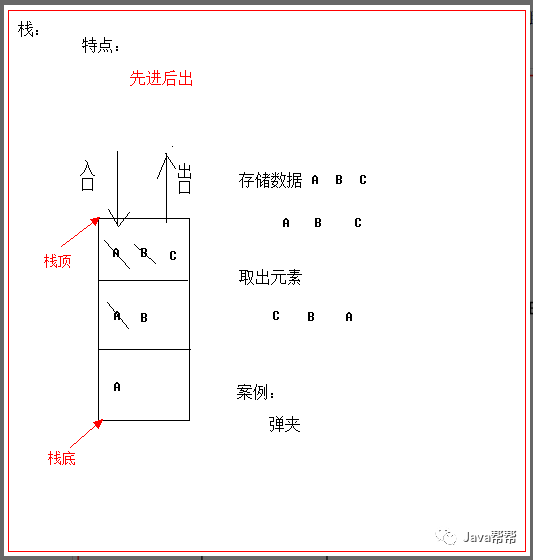• 队列，采用该结构的集合，对元素的存取有如下的特点：

• 先进先出（即，存进去的元素，要在后它前面的元素依次取出后，才能取出该元素）。例如，安检。排成一列，每个人依次检查，只有前面的人全部检查完毕后，才能排到当前的人进行检查。

• 队列的入口、出口各占一侧。例如，下图中的左侧为入口，右侧为出口。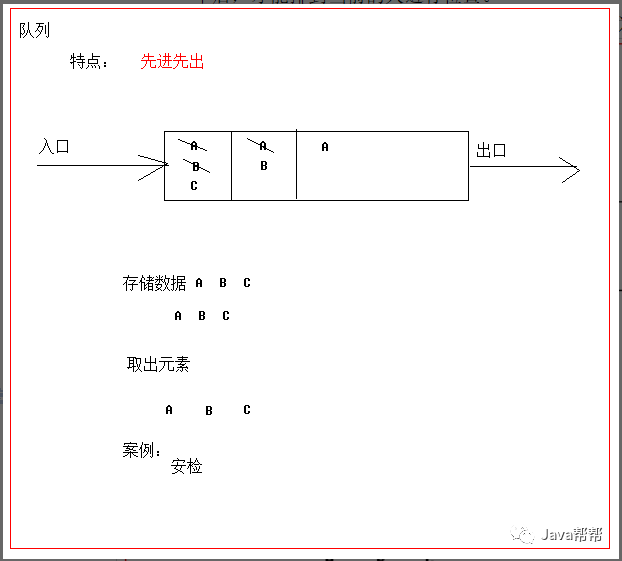• 数组，采用该结构的集合，对元素的存取有如下的特点：

• 查找元素快：通过索引，可以快速访问指定位置的元素

• 增删元素慢：

• 指定索引位置增加元素：需要创建一个新数组，将指定新元素存储在指定索引位置，再把原数组元素根据索引，复制到新数组对应索引的位置。如下图

• 指定索引位置删除元素：需要创建一个新数组，把原数组元素根据索引，复制到新数组对应索引的位置，原数组中指定索引位置元素不复制到新数组中。如下图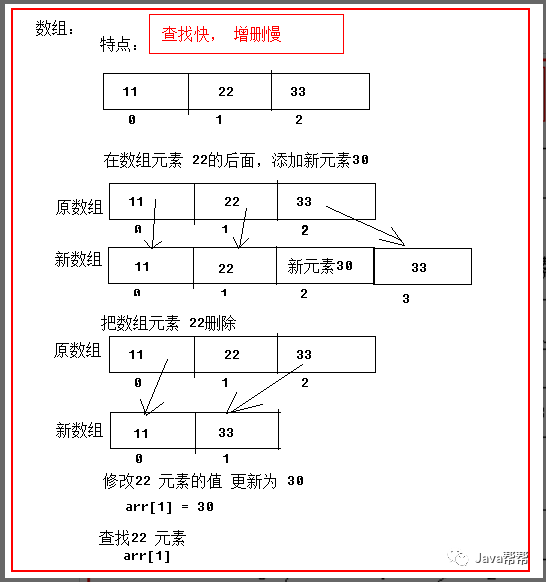• 链表，采用该结构的集合，对元素的存取有如下的特点：

• 多个节点之间，通过地址进行连接。例如，多个人手拉手，每个人使用自己的右手拉住下个人的左手，依次类推，这样多个人就连在一起了。

• 查找元素慢：想查找某个元素，需要通过连接的节点，依次向后查找指定元素

• 增删元素快：

• 增加元素：操作如左图，只需要修改连接下个元素的地址即可。

• 删除元素：操作如右图，只需要修改连接下个元素的地址即可。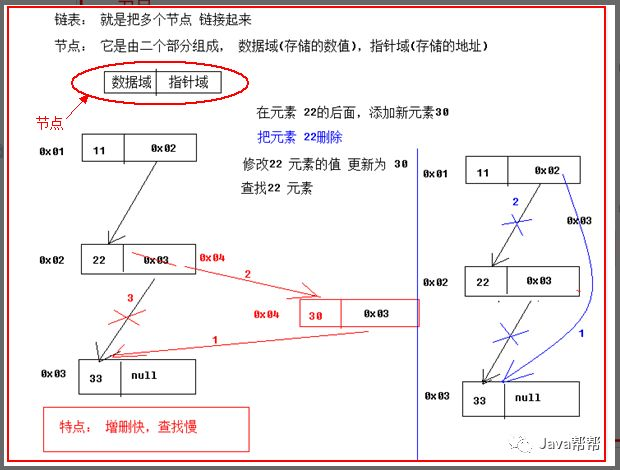## 2.3 List接口常用方法

void add(int index, E element)  //指定索引添加元素

E remove(int index)     //移除指定索引处元素

E get(int index)     //获取指定索引元素

E set(int index, E element)   //修改指定索引元素

List<E> subList(int fromIndex, int toIndex) //截取指定索引子集

int indexOf(Object o)    //返回指定元素索引位置

import java.util.ArrayList;

import java.util.List;

/*

* List 方法

*

* void add(int index, E element) //指定索引添加元素

*/

public class Demo {

public static void main(String[] args) {

//fun3();

//fun2();

//fun();

}

private static void fun3() {

List <String > al = new ArrayList<>();

System.out.println(al);

al.set(2, "6666");

System.out.println(al);

List<String> subList = al.subList(1, 4);

System.out.println(subList);

int indexOf = subList.indexOf("444");

System.out.println(indexOf);

}

private static void fun2() {

List <Integer > al = new ArrayList<>();

al.remove(2);

System.out.println(al);

}

private static void fun() {

List <String > al = new ArrayList<>();

System.out.println(al);

al.remove(6);

System.out.println(al);

}

}

ListIterator<E> listIterator()   注意：用于应对并发修改异常的返回迭代器方法与迭代器

import java.util.ArrayList;

import java.util.Collection;

import java.util.Iterator;

import java.util.List;

import java.util.ListIterator;

/*

* ListIterator : Iterator 子接口.

*

* Collection 是得不到ListIterator , 因为 List 专属的.

*

*/

public class Demo2 {

public static void main(String[] args) {

//fun();

List c  = new ArrayList<>();

ListIterator li  = c.listIterator();

while (li.hasNext()) {

Object object = (Object) li.next();

if (object.equals("白骨精")) {

}

}

System.out.println(c);

ListIterator listIterator = c.listIterator();

listIterator.next();

System.out.println(listIterator.hasPrevious());

}

private static void fun() {

Collection c  = new ArrayList<>();

//遍历 集合, 如果 包含 白骨精 ,你就添加一个 白龙马.

Iterator iterator = c.iterator();

while (iterator.hasNext()) {

Object object = (Object) iterator.next();

if (object.equals("白骨精")) {

}

}

System.out.println(c);

}

}

## 2.4 具体子类介绍

### 2.4.1 ArrayList

ArrayList底层数据结构是数组结构。线程不安全的，所以运行速度快，ArrayList的出现替代了Vector。增删慢,查找快，由于日常开发中使用最多的功能为查询数据，遍历数据，所以ArrayList是最常用的集合。目前市面上许多程序员开发时并不严谨，非常随意地使用ArrayList完成任何需求，这种用法是不提倡的。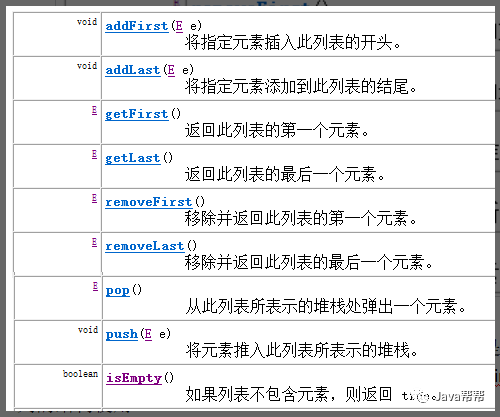//添加元素

//获取元素

//删除元素

}

### 2.4.3 Vector

Vector：我们可以将其理解为版本旧的、安全的、效率低的ArrayList，Vector中提供了一个独特的取出方式，就是枚举Enumeration。此接口Enumeration的功能与 Iterator 接口的功能是类似的。

public E elementAt(int index)  / get(index )

public E firstElement()

public E lastElement()

public void setElementAt(E obj, int index) set(index,obj)

public void removeElementAt(int index)及其他删除 remove(index)

public Enumeration<E> elements()

import java.util.Enumeration;

import java.util.Vector;

/*

* Vector :  数组结构,单线程的 ArrayList.

*/

public class Demo2 {

public static void main(String[] args) {

Vector<String > vector = new Vector<>();

// 获取 枚举.

Enumeration<String> elements = vector.elements();

while (elements.hasMoreElements()) {

String nextElement = elements.nextElement();

System.out.println(nextElement);

}

}

}

# 第3章 Set接口

## 3.1 HashSet集合介绍

HashSet集合，采用哈希表结构存储数据，保证元素唯一性的方式依赖于：hashCode()与equals()方法。

## 3.2 HashSet集合存储数据的结构（哈希表）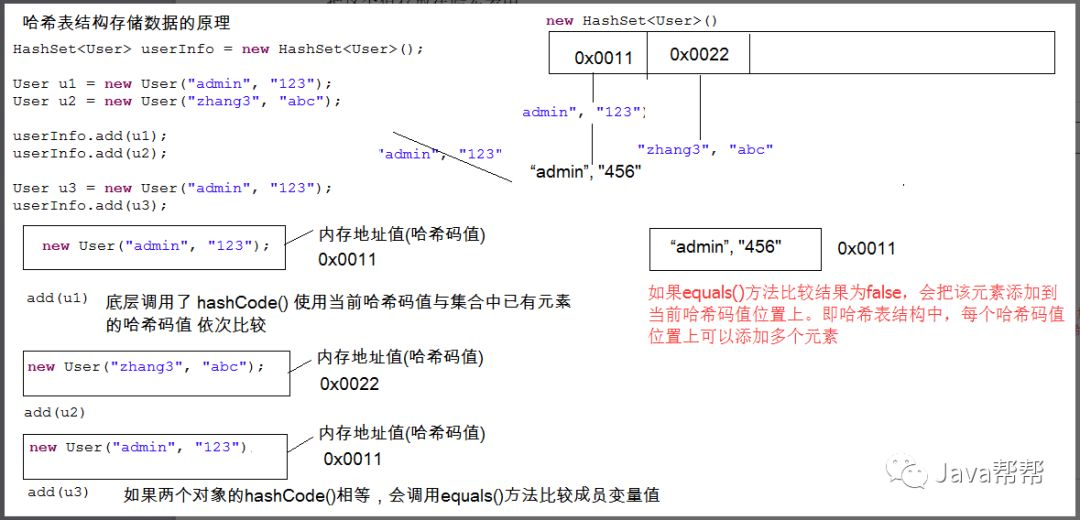## 3.3 HashSet存储JavaAPI中的类型元素

• 创建HashSet集合，存储String对象。

public class HashSetDemo {

public static void main(String[] args) {

//创建HashSet对象

HashSet<String> hs = new HashSet<String>();

//给集合中添加自定义对象

//取出集合中的每个元素

Iterator<String> it = hs.iterator();

while(it.hasNext()){

String s = it.next();

System.out.println(s);

}

}

}

wangwu

lisi

zhangsan

## 3.4 HashSet存储自定义类型元素

• 创建自定义对象Student

public class Student {

private String name;

private int age;

public Student(String name, int age) {

super();

this.name = name;

this.age = age;

}

public String getName() {

return name;

}

public void setName(String name) {

this.name = name;

}

public int getAge() {

return age;

}

public void setAge(int age) {

this.age = age;

}

@Override

public String toString() {

return "Student [name=" + name + ", age=" + age + "]";

}

@Override

public int hashCode() {

final int prime = 31;

int result = 1;

result = prime * result + age;

result = prime * result + ((name == null) ? 0 : name.hashCode());

return result;

}

@Override

public boolean equals(Object obj) {

if (this == obj)

return true;

if(!(obj instanceof Student)){

System.out.println("类型错误");

return false;

}

Student other = (Student) obj;

return this.age ==  other.age && this.name.equals(other.name);

}

}

• 创建HashSet集合，存储Student对象。

public class HashSetDemo {

public static void main(String[] args) {

//创建HashSet对象

HashSet hs = new HashSet();

//给集合中添加自定义对象

//取出集合中的每个元素

Iterator it = hs.iterator();

while(it.hasNext()){

Student s = (Student)it.next();

System.out.println(s);

}

}

}

Student [name=lisi, age=22]

Student [name=zhangsan, age=21]

Student [name=wangwu, age=23]

## 3.5 判断元素唯一原理

### 3.5.1 ArrayList的contains方法判断元素是否重复原理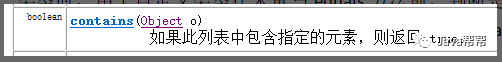ArrayList的contains方法会使用调用方法时，传入的元素的equals方法依次与集合中的旧元素所比较，从而根据返回的布尔值判断是否有重复元素。此时，当ArrayList存放自定义类型时，由于自定义类型在未重写equals方法前，判断是否重复的依据是地址值，所以如果想根据内容判断是否为重复元素，需要重写元素的equals方法。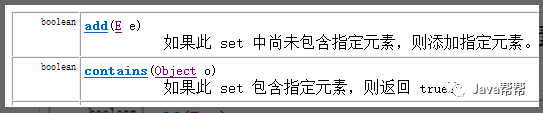Set集合不能存放重复元素，其添加方法在添加时会判断是否有重复元素，有重复不添加，没重复则添加。

HashSet集合由于是无序的，其判断唯一的依据是元素类型的hashCode与equals方法的返回结果。规则如下：

• 如果不同，说明是不同元素，添加到集合。

• 如果相同，再判断equals比较结果。返回true则相同元素；返回false则不同元素，添加到集合。

hashCode方法重写规则：将该对象的各个属性值hashCode相加即是整个对象的HashCode值。如果是基本类型，类似int，则直接返回int值就是该属性的hash值，如果是引用类型，类似String，就调用该成员变量的hashCode方法返回该成员变量hash值。这样可以根据对象的内容返回hashCode值，从而可以根据hashCode判断元素是否唯一。

public class Student {

String name ;

int age;

public Student() {

super();

// TODO Auto-generated constructor stub

}

public Student(String name, int age) {

super();

this.name = name;

this.age = age;

}

@Override

public String toString() {

return "Student [name=" + name + ", age=" + age + "]";

}

// 想要保证 对象的唯一性, 必须重写 equals hashCode();

// (老王, 60 )  , (金莲 ,28 )

// hashCode 而是通过 属性的值计算出来的hashCode

@Override

public int hashCode() {

final int prime = 31;

int result = 1;

result = prime * result + age;  // 91     59

result = prime * result + ((name == null) ? 0 : name.hashCode());

return result;

}

@Override

public boolean equals(Object obj) {

if (this == obj) // 当前对象与 传参对象  地址 相同 .返回true .

return true;

if (obj == null) //传参对象 为null, 返回 false .

return false;

//当前对象与 传参对象 不一样, 返回false .

if (this.getClass() != obj.getClass()) // getClass() 获取类型

return false;

// 强制转换 .

Student other = (Student) obj;

if (age != other.age) //比较年龄

return false;

if (name == null) { //  比较名字, 我没名,你有名, 返回false

if (other.name != null)

return false;

} else if (!name.equals(other.name)) //  姓名不一样,返回false .

return false;

return true;

}

}

import java.util.HashSet;

/*

* Set : 不包含重复元素的集合.无序     常用 其子类 HashSet .

*

* 你想要使用HashSet保存自定义类型的元素,必须通过重写hashCodeequals方法来保证对象的唯一性.

* 记住: 重写就好使,不重写就挂了. alt + shift + s  --> h

*/

public class Demo {

public static void main(String[] args) {

// fun();

HashSet<Student> hashSet = new HashSet<>();

Student student = new Student("金莲", 28);

Student student2 = new Student("小明", 15);

Student student3 = new Student("韩梅梅", 16);

Student student4 = new Student("李雷", 18);

Student student5 = new Student("李雷", 18);

Student student6 = new Student("李雷", 18);

Student student7 = new Student("李雷", 18);  // 属性值 全一样, 说明 是同一个对象.

//  打印 出几个同学?

System.out.println(hashSet);

}

private static void fun() {

HashSet<String> hashSet = new HashSet<>();

// 添加元素

System.out.println(hashSet);

}

}

## 3.6 Hashset练习

### 3.6.1 编写一个程序，获取10个1至20的随机数,要求随机数不能重复

import java.util.HashSet;

import java.util.Iterator;

import java.util.Random;

/*

* 需求：编写一个程序，获取10120的随机数,要求随机数不能重复。

* 并把最终的随机数输出到控制台。

*/

public class Test {

public static void main(String[] args) {

// 创建Random 对象

Random random = new Random();

//创建集合

HashSet<Integer> hashSet = new HashSet<>();

int count = 0;

// 循环获取,循环添加 , 集合的个数做为条件.

while (hashSet.size() < 10) {

count ++; // 计数

// 调用Random 获取随机数

int i  = random.nextInt(20) + 1;

// 添加到集合 , HashSet , 无序,唯一的.

}

System.out.println(count);

System.out.println("添加完毕,遍历集合");

// 遍历 hashSet

Iterator<Integer> iterator = hashSet.iterator();

while (iterator.hasNext()) {

Integer integer = (Integer) iterator.next();

System.out.print(integer +" ");

}

}

}

### 3.6.2 将集合中的重复元素去掉(自定义类 )

public class Student {

String name;

int age;

public Student(String name, int age) {

super();

this.name = name;

this.age = age;

}

@Override

public String toString() {

return " Student 姓名=" + name + ", 年龄=" + age ;

}

@Override

public int hashCode() {

final int prime = 31;

int result = 1;

result = prime * result + age;

result = prime * result + ((name == null) ? 0 : name.hashCode());

return result;

}

@Override

public boolean equals(Object obj) {

if (this == obj)

return true;

if (obj == null)

return false;

if (getClass() != obj.getClass())

return false;

Student other = (Student) obj;

if (age != other.age)

return false;

if (name == null) {

if (other.name != null)

return false;

} else if (!name.equals(other.name))

return false;

return true;

}

// 重写hashCode equals

}

import java.util.ArrayList;

import java.util.HashSet;

/*

* 将集合中的重复元素去掉(自定义类 )

ArrayList<Student> list = new ArrayList<>();

Student student = new Student("金莲", 28);

Student student2 = new Student("小明", 15);

Student student3 = new Student("韩梅梅", 16);

Student student4 = new Student("李雷", 18);

Student student5 = new Student("李雷", 18);

Student student6 = new Student("李雷", 18);

*/

public class Test2 {

public static void main(String[] args) {

ArrayList<Student> list = new ArrayList<>();

Student student = new Student("金莲", 28);

Student student2 = new Student("小明", 15);

Student student3 = new Student("韩梅梅", 16);

Student student4 = new Student("李雷", 18);

Student student5 = new Student("李雷", 18);

Student student6 = new Student("李雷", 18);

System.out.println(list);

//创建hashSet

HashSet<Student> hashSet = new HashSet<>();

for (Student s  : list) {

}

System.out.println(hashSet);

}

}

# 第4章 集合综合案例

• 准备牌：

• 发牌：

• 看牌：

##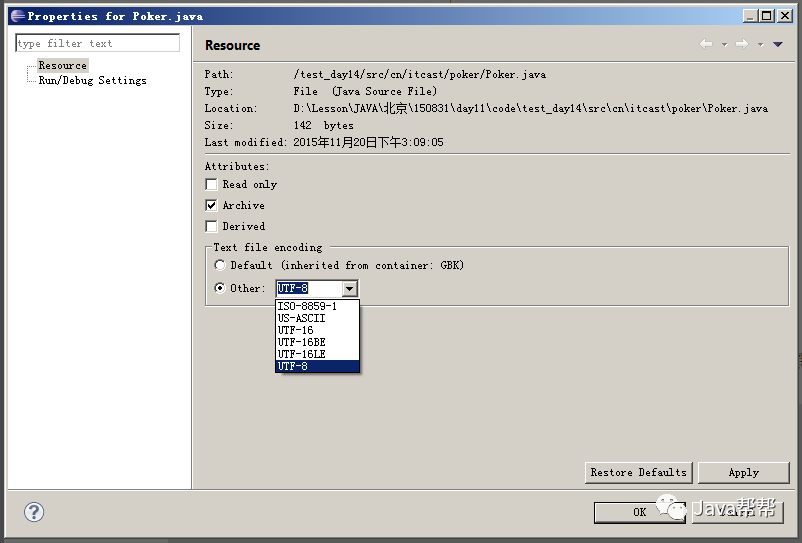public class Poker {

public static void main(String[] args) {

//♠♥♦♣

//准备牌

ArrayList<String> poker = new ArrayList<String>();

//花色

ArrayList<String> color = new ArrayList<String>();

//数字

ArrayList<String> number = new ArrayList<String>();

for (int i = 2; i <= 10; i++) {

}

//完成新牌

for (String thisColor : color) {

for (String thisNumber : number) {

String thisCard = thisColor + thisNumber;

}

}

//洗牌

Collections.shuffle(poker);

//发牌

//玩家1

ArrayList<String> player1 = new ArrayList<String>();

//玩家2

ArrayList<String> player2 = new ArrayList<String>();

//玩家3

ArrayList<String> player3 = new ArrayList<String>();

//底牌

ArrayList<String> secretCards = new ArrayList<String>();

for (int i = 0; i < poker.size(); i++) {

if(i>=51) {

//最后三张发给底牌

}else {

//剩余牌通过对3取模依次摸牌

if(i%3==0) {

}else if(i%3==1) {

}else {

}

}

}

//看牌

System.out.println(player1);

System.out.println(player2);

System.out.println(player3);

System.out.println(secretCards);

}

}

• 最后发到三个人手中的牌是无序的，在明天学习完Map集合后，我们提供一个排序的解决方案。

# 第5章 Collection知识点总结

• Collection:

|- List 可以存储重复元素,有序的(元素存取顺序)

|- ArrayList

|- Set 不能存储重复元素,无序的(元素存取顺序)

|- HashSet

• Collection方法：

• void clear() 清空集合中所有的元素

• boolean remove(Object o) 把给定的对象在当前集合中删除

• boolean contains(Object o) 判断当前集合中是否包含给定的对象

• boolean isEmpty() 判断当前集合是否为空

• Iterator iterator() 迭代器，用来遍历集合中的元素的

• int size() 返回集合中元素的个数

• Object[] toArray() 把集合中的元素，存储到数组中

• Iterator :  迭代器

• Object next()返回迭代的下一个元素

• boolean hasNext()如果仍有元素可以迭代，则返回 true。

• List与Set集合的区别？

List:

它是一个有序的集合(元素存与取的顺序相同)

它可以存储重复的元素

Set:

它是一个无序的集合(元素存与取的顺序可能不同)

它不能存储重复的元素

• List集合中的特有方法

• void add(int index, Object element) 将指定的元素，添加到该集合中的指定位置上

• Object get(int index)返回集合中指定位置的元素。

• Object remove(int index) 移除列表中指定位置的元素, 返回的是被移除的元素

• Object set(int index, Object element)用指定元素替换集合中指定位置的元素,返回值的更新前的元素

• ArrayList:

底层数据结构是数组，查询快，增删慢

线程不安全，效率高

底层数据结构是链表，查询慢，增删快

线程不安全，效率高

• 泛型： 用来约束数据的数据类型

• 泛型的格式：

<数据类型>

泛型可以使用在 类，接口，方法，变量上

• 泛型的好处

A:提高了程序的安全性

B:将运行期遇到的问题转移到了编译期

C:省去了类型强转的麻烦

• 增强for

简化数组和Collection集合的遍历

格式：

for(元素数据类型 变量 : 数组或者Collection集合) {

使用变量即可，该变量就是元素

}

好处：简化遍历

• HashSet:

元素唯一不能重复

底层结构是 哈希表结构

元素的存与取的顺序不能保证一致

如何保证元素的唯一的？

重写hashCode() 与 equals()方法

元素唯一不能重复

底层结构是 哈希表结构 + 链表结构

元素的存与取的顺序一致

# 第6章 本日自习作业：

## 6.1 知识点相关题

1. 知识点：自定义类  迭代器  泛型  增强for

1) 按以下步骤编写代码：

a) 定义类：Cat，包含以下成员：

成员属性(私有)：

名称：

颜色：

年龄：

构造方法：

无参

全参

成员方法：

1).get/set方法；

2).重写toString()方法；内部打印所有属性的值；

b) 在main()方法中，按以下步骤编写代码：

• 向集合中添加以下cat对象：

“波斯猫”，“白色”，2

“折耳猫”，“灰色”，1

“加菲猫”，“红色”，3

“机器猫”，“蓝色”，5

1） 使用普通for循环

2） 迭代器(需要指定泛型)

3） 增强for三种方式

### 6.1.1 泛型的好处是什么？泛型用于什么上？

1.提高了程序的安全性

2.将运行期遇到的问题转移到了编译期

3.省去了类型强转的麻烦

泛型的常见应用：

1.泛型类

2.泛型方法

3.泛型接口

### 6.1.2 请简述List<? extends T>和List<? super T>之间有什么区别？

List<? extends T> ：向下限制

List<? super T> ：向上限制

? extends T ： 代表接收的泛型类型为T类型或T子类类型

? super T ：代表接收的泛型类型为T类型或T父类类型

### 6.1.4 请编写程序，存储3个手机对象到ArrayList集合中

a) 使用迭代器进行遍历,要有泛型

b) 打印出三个手机对象的信息，比如颜色，品牌。

### 6.1.16 在括号中选择对应结构的解释，什么是数据结构(),什么是数组结构(),什么是链表结构(),什么是队列结构(),什么是栈结构()

A:数据存储和组织方式

B:容器先进后出规则

C:容器先进先出的规则

D:每个元素指向下一个元素

E:一块连续的存储区域

 答案说明：数组结构:一块连续的存储区域，查询速度快，添加速度慢链表结构:每个元素指向下一个元素，添加快，查询慢队列结构:容器先进先出的规则栈结构:容器先进后出规则

### 6.1.17 给定一个字符串数组，数组内容为：{"我","爱","编程","但是","我","更","爱","IPHONE"}创建一个集合，这个集合存储上面字符串数组里面的元素，并且不存储重复的，此时的集合打印效果为：[更, 爱, IPHONE, 我, 编程, 但是]，遍历集合，将集合中元素是IPHONE的元素删除，过后添加一个新的元素，元素为MJ，最终的集合的打印效果为：[更,爱, 我, 编程, MJ, 但是]

 package day08_Test基础练习题;import java.util.HashSet;public class Test005 {public static void main(String[] args) {HashSet list = new HashSet<>();String[] str = {"我","爱","编程","但是","我","更","爱","IPHONE"};for (int i = 0; i < str.length; i++) {list.add(str[i]);}System.out.println(list);for (String string : str) {if(string.equals("IPHONE")){list.remove(string);//添加元素list.add("MJ");}}System.out.println("操作过后的元素为：");System.out.println(list);}}

### 6.1.18 创建Person类并测试

1.编写Person类，有age属性(数据类型是int)和name属性(数据类型是String),且都被private修饰，提供get/set方法，不重写equals方法和hashCode方法

2.编写Test类，在Test类的main方法中定义一个ArrayList集合，集合内存放的元素的数据类型是Person类

• 分别创建三个对象，对象p1的年龄为10，名字为李四；对象p2的年龄为20，名字为王五；对象p3的年龄为30，名字为小强

• 将三个对象依次添加到定义的集合中

• 创建一个对象p4,此对象的年龄为30，名字为小强

• 调用集合的contains方法，将p4作为参数传递进去，查看打印的是true还是false

• 如果打印为false，那么在Person类中重写hashCode和equals方法

• 查看打印的结果是否为true

 Person类：package day08_Test基础练习题;public class Person {private int age;private String name;public int getAge() {return age;}public void setAge(int age) {this.age = age;}public String getName() {return name;}public void setName(String name) {this.name = name;}public Person(int age, String name) {super();this.age = age;this.name = name;}public Person() {super();// TODO Auto-generated constructor stub}@Overridepublic int hashCode() {final int prime = 31;int result = 1;result = prime * result + age;result = prime * result + ((name == null) ? 0 : name.hashCode());return result;}@Overridepublic boolean equals(Object obj) {if (this == obj)return true;if (obj == null)return<